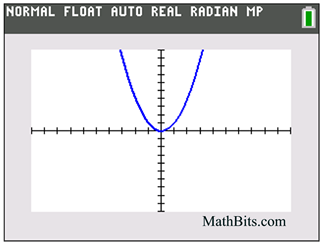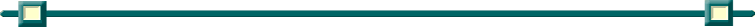Graphing Continuous vs Discrete Functions

 A continuous function allows the x-values to be ANY points in the interval, including fractions, decimals, and irrational values. A discrete function allows the x-values to be only certain points in the interval, usually only integers or whole numbers.: Graph the continuous function: y = x2 for all Reals. • Enter the function into "y=". • Choose the window. ZOOM #6 (standard) • GraphNotice that all of the visable points are connected with a continual drawing. Graph the discrete function: y = x2 for all Integers. • We cannot limit the point choices in "y=". We need a different approach, the scatter plot. • If your viewing window is the standard 10x10 window, you will have to determine which points will be visible in this window: (x = -3, -2, -1, 0, 1, 2, 3) • STAT - Edit - enter x (L1) and y (L2) values. • Stat-Plot - Plot 1 - turn Plot "On" - Choose "Type" scatter plot (first icon) - Xlist: L1 - Ylist: L2 - choose any Mark. • Choose ZOOM #9 (statistics) • GraphNotice that only the integer values are plotted. The points are NOT connected.Finding Your Way Around TABLE of  CONTENTS